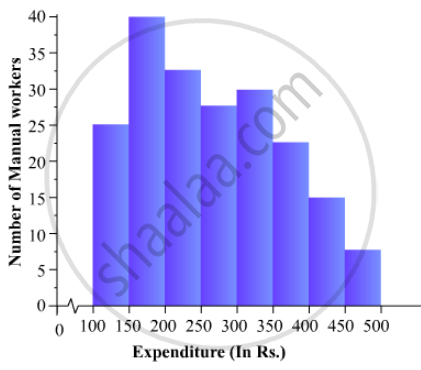# The Following is the Distribution of Total Household Expenditure (In Rs.) of Manual Worker in a City:Draw a Histogram and a Frequency Polygon Representing the Above Data. - Mathematics

The following is the distribution of total household expenditure (in Rs.) of manual worker in a city:

 Expenditure(in Rs): 100-150 150-200 200-250 250-300 300-350 350-400 400-450 450-500 No. of manual workers: 25 40 33 28 30 22 16 8

Draw a histogram and a frequency polygon representing the above data.

#### Solution

To represent the given data by a histogram, we first draw horizontal and vertical axes. Let us consider that the horizontal and vertical axes represent the class-limits and the frequencies of the class-intervals respectively.

The given data is a continuous grouped frequency distribution with equal class-intervals. Construct rectangles with class-intervals as bases and respective frequencies as heights. It should be noted that the scale for horizontal axis may not be same as the scale for vertical axis. To draw the frequency polygon of the given data using histogram, obtain the mid-points of the upper horizontal side of each rectangle and then join these mid-points of the adjacent rectangles of the histogram by line segments. Obtain the mid-points of two class-intervals of 0 frequencies, i.e. on the horizontal axis, one adjacent to the first, on its left and one adjacent to the last, on its right. These class-intervals are known as imagined class-intervals. Complete the polygon by joining the mid-points of first and last class-intervals to the mid-points of imagined class-intervals adjacent to them. Let us take one vertical division is equal to 5 workers.

The heights of the different rectangles are as follows:

1. The height of the rectangle corresponding to the class-interval 100-150 is 25/5=5 big divisions.

2. The height of the rectangle corresponding to the class-interval 150-200 is 40/5=8 big divisions.

3. The height of the rectangle corresponding to the class-interval 200-250 is  33/5 = 6.6 big divisions.

4. The height of the rectangle corresponding to the class-interval 250-300 is  28/5 = 5.6  big  divisions.

5. The height of the rectangle corresponding to the class-interval 300-350 is   30/5 =6  big divisions.

6. The height of the rectangle corresponding to the class-interval 350-400 is  22/5 = 4.4  big divisions.

7. The height of the rectangle corresponding to the class-interval 400-450 is  16/5 = 3.2  big division.

8. The height of the rectangle corresponding to the class-interval 450-500 is  8/5 = 1.6 big divisions.

The histogram of the given data is as follows:Concept: Graphical Representation of Data
Is there an error in this question or solution?

#### APPEARS IN

RD Sharma Mathematics for Class 9
Chapter 23 Graphical Representation of Statistical Data
Exercise 23.3 | Q 5 | Page 42

Share